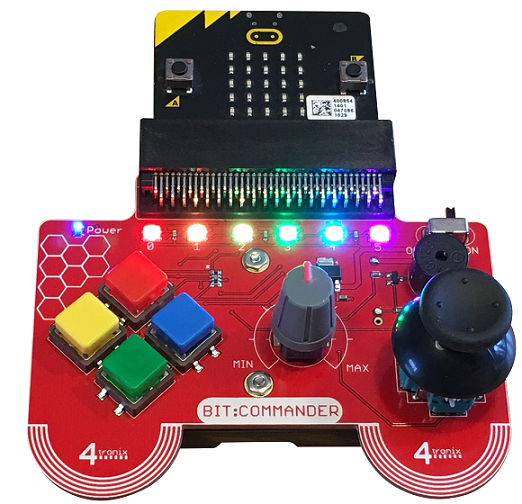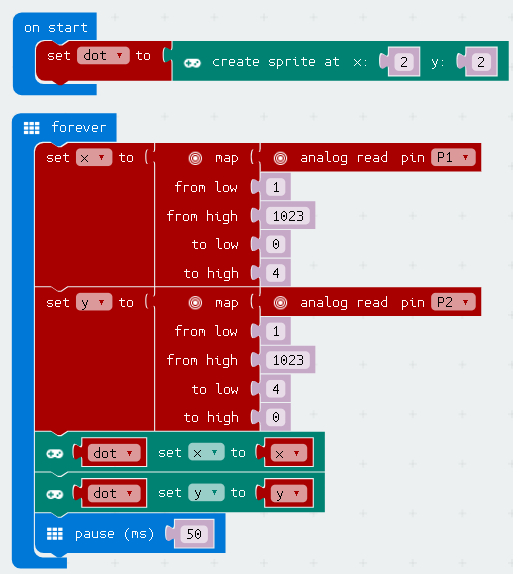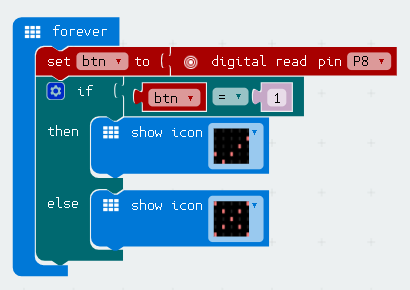# BBC micro:bit Bit:Commander - The Joystick

## Introduction

The joystick on the Bit:Commander is exactly the same type as you would find on a standard game controller, what you might call thumbsticks. These joysticks are effectively two potentiometers, one for the x axis and one for the y axis. Movement on each axis effects the reading on each of the potentiometers, allowing you to determine the direction and distance that the stick was moved. This means that you can use the joystick readings to make variable changes to parts of your program or circuit, like moving faster if the stick is pushed further forwards. Pressing the stick closes a pushbutton that can also be read.## Programming

You use analog read blocks to get a reading from the joystick. You get a reading from 1 to 1023. In this test program, a sprite is created. The analog readings on the joystick axes are mapped to a smaller range (0 to 4). The sprite is moved around the screen using the joystick.Notice that the range for the y axis has the low and high numbers in the reverse order. If you swap them around, you will have to move up to move down and down to move up.

### JavaScript

```let y = 0
let x = 0
let dot: game.LedSprite = null
dot = game.createSprite(2, 2)
basic.forever(() => {
x = pins.map(
1,
1023,
0,
4
)
y = pins.map(
1,
1023,
4,
0
)
dot.set(LedSpriteProperty.X, x)
dot.set(LedSpriteProperty.Y, y)
basic.pause(50)
})
```

Like most thumbsticks, the stick is also a pushbutton. You use digital read blocks to read the button state from pin 8. This program detects whether or not the stick is being pressed. It displays a tick when the stick is pressed and a cross when it is not.### JavaScript

```let btn = 0
basic.forever(() => {